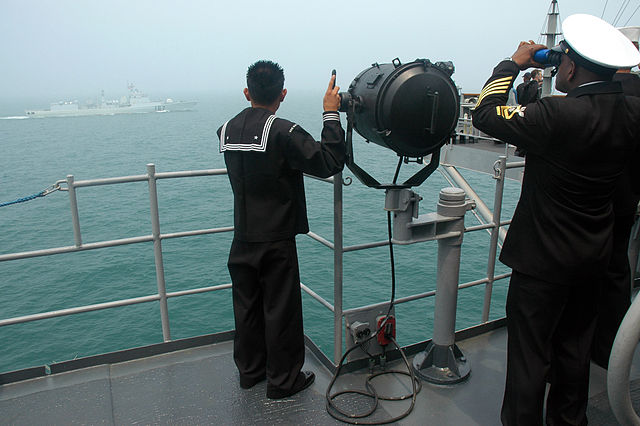# Far, far away?If you have not done the previous set, I recommend you do it first.

Once we have the concept of synchronized clocks and a definition of how to relate the time coordinates of observers at different points we can define a distance. Let's choose a region of space with a family of observers moving through it, all with clocks synchronized by sending light pulses back and forth. Before when dealing with time we used the variable $F$ for Finn's time and $A$ for Aaron's time. Since we now have synchronized clocks, we can define a variable $t$ as the coordinate time which is a valid, well defined coordinate everywhere in the region of interest.

Consider the scenario where Finn and Aaron are communicating with light pulses. At time $t$$_1$, Finn sends a light pulse to Arron, who reflects is back, and is received by Finn at time $t$$_2$. Which of these is a possible definition for the distance $x$ between Finn and Aaron?

×